HOME / Applications / RLC Bandpass Filter

# RLC Bandpass Filter

Used Tools:## Description

An RLC circuit is an electrical circuit formed of a number of resistors, inductors and capacitors. There are multiple applications for this type of circuits. Some of the most important ones are oscillators, tuners of radio receivers and television sets and of course filters. RLC circuits are used  to create band-pass and band-stop filters as well.

The RLC filter is normally called a second order circuit which means that the circuit parameters such as voltage and current in can be described by a differential equation of second-order. A problem that occurs in designing such filters is the resistance shown by the inductor. As the inductors are fabricated by coils of wires they do have an undesirable resistance that could have a significant effect on the performance of the circuit. That is why it is always a good idea to do a field analysis of such configurations during the design. HFWorks enables the designer of simulating such circuits either by specifying the values of the lumped elements used in the circuits or by modeling the actual coil.

This tutorial focuses on an RLC filter made up of lumped elements, along with microstrip lines, profiting of HFWorks' ability to mix both types of simulations: circuit and 3D models together in a single study. The band-pass filter operates at 1 GHz with a bandwidth of 10%.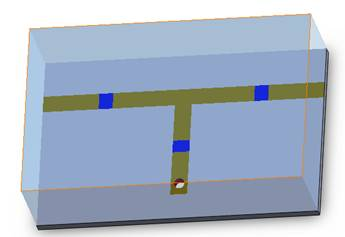Figure 1 - 3D view of an RLC filter

## Simulation

To simulate the behavior of this filter (insertion and return loss at the desired frequency band, input and output matching), we will create a Scattering Parameters study, and specify the relevant frequency band at which the filter operates (in our case 21 frequencies uniformly distributed from 1.8 GHz to 3.8 GHz).

The simulated study provides multiple choices and plot  options to adjust the outputted results to the user's intention.

## Solids and Materials

The microstrip lines (conductor and ground metals) are supposed to be perfect electric conductors built on a Mat1 substrate. RLC components are modeled on three shields and they have been chosen the following inductance and capacitance values: 127 nH and 0.2 pF respectively for the shields close to ports. The parallel shield has these values: 0.726 nH and 34.9 pF being implemented as parallel RLC circuit.

The ports are applied to the lateral faces of the substrate (the sides of the horizontal microstrip line with the two RLC shields) and the air box. This way, the simulation considers the electric field's distribution in the air and the radiation boundaries would give results more conveniently to quasi-TEM theory of waves' transmission over a microstrip line.

## Meshing

Since the signal's path implicates the port and the in signal surfaces, the mesh has to be concentrated on these parts. Meshing these surfaces helps the solver refine its precision on the eddy parts, and take the path's shape into account.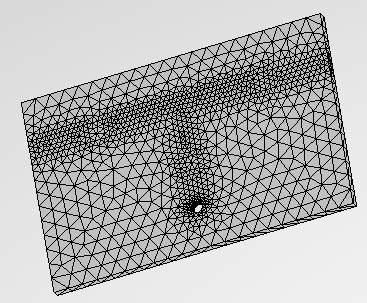Figure 2 - Mesh of the dipole antenna

## Results

Various 3D and 2D plots are available to exploit, depending on the nature of the task and on which parameter the user is interested in. As we are dealing with a filter simulation, plotting the insertion and return losses sounds like an intuitive task. The following figure shows both the insertion and return losses of the considered filter: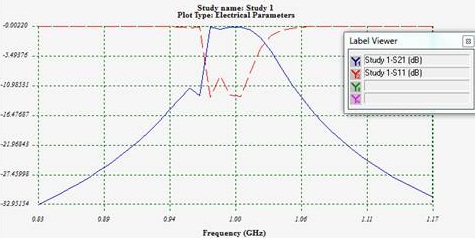Figure 3 - Insertion and return loss of the filter around 1 GHz

The insertion loss appears to be very low within the frequency band around 1 GHz and gives great isolation below 0.94 GHz and above 1.06 GHz. Plotting the return loss on a Smith chart plot is more relevant when we deal with matching issues. For this purpose, the following figure illustrates the S11 curve along with a red marker showing the return loss at 1 GHz.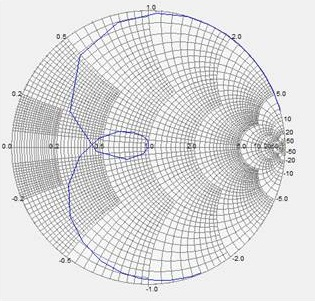Figure 4 - Variations of reflection coefficient at the filter's port

The electric field distribution at 1 GHz has been spotted on the following figure, we can see clearly that the electromagnetic wave goes through the circuit and reaches the second port.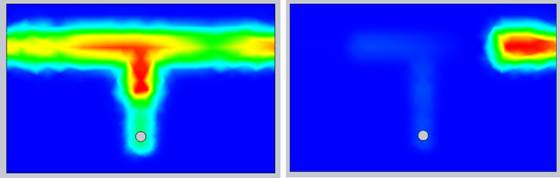Figure 5 - Electric field vector distribution on the circuit at the aimed frequency (1 GHz and 0.83 GHz)